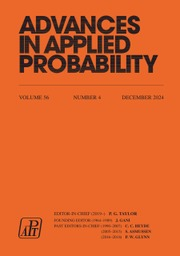Home
Hostname: page-component-56f9d74cfd-w6k7h Total loading time: 0.241 Render date: 2022-06-27T01:42:58.418Z Has data issue: true Feature Flags: { "shouldUseShareProductTool": true, "shouldUseHypothesis": true, "isUnsiloEnabled": true, "useRatesEcommerce": false, "useNewApi": true }Advances in Applied Probability

# Complications with stochastic volatility models

Published online by Cambridge University Press:  01 July 2016

## Abstract

We show a class of stock price models with stochastic volatility for which the most natural candidates for martingale measures are only strictly local martingale measures, contrary to what is usually assumed in the finance literature. We also show the existence of equivalent martingale measures, and provide one explicit example.

## MSC classification

Type
General Applied Probability
Information
Advances in Applied Probability , March 1998 , pp. 256 - 268

## Access options

Get access to the full version of this content by using one of the access options below. (Log in options will check for institutional or personal access. Content may require purchase if you do not have access.)

## References

Ansel, J.-P. and Stricker, C. (1993). Unicité et existence de la loi minimale. In Séminaire de Probabilités XXVII (Lecture Notes in Mathematics 1557). Springer, Berlin, pp. 2229.CrossRefGoogle Scholar
Dalang, R., Morton, A. and Willinger, W. (1990). Equivalent martingale measures and no-arbitrage in stochastic securities market models. Stoch. Stoch. Rep. 29, 185201.CrossRefGoogle Scholar
Delbaen, F. (1992). Representing martingale measures when asset prices are continuous and bounded. Math. Finance 2, 107130.CrossRefGoogle Scholar
Delbaen, F. and Schachermayer, W. (1994a). Arbitrage and free lunch with bounded risk for unbounded continuous processes. Math. Finance 4, 343348.CrossRefGoogle Scholar
Delbaen, F. and Schachermayer, W. (1994b). A general version of the fundamental theorem of asset pricing. Math. Ann. 300, 463520.CrossRefGoogle Scholar
Delbaen, F. and Schachermayer, W. (1995). The no-arbitrage property under a change of numéraire. Stoch. Stoch. Rep. 53, 213226.Google Scholar
Dupire, B. (1992). Arbitrage pricing with stochastic volatility. Preprint.Google Scholar
Eisenberg, L. and Jarrow, R. (1994). Option pricing with random volatilities in complete markets. Rev. Quant. Finance and Accounting 4, 517.CrossRefGoogle Scholar
Elliot, R. (1982). Stochastic Calculus and Applications. Springer, New York.Google Scholar
Harrison, M. and Kreps, D. (1979). Martingales and arbitrage in multiperiod security markets. J. Econ. Theory 20, 381408.CrossRefGoogle Scholar
Harrison, M. and Pliska, S. (1981). Martingales and stochastic integrals in the theory of continuous trading. Stoch. Proc. Appl. 11, 215260.CrossRefGoogle Scholar
Heath, D. and Sin, C. (1996). A note on local martingale measures and the fundamental theorem of asset pricing. Preprint, Cornell University.Google Scholar
Heston, S. (1993). A closed form solution for options with stochastic volatility with applications to bond and currency options. Rev. Financial Studies 6, 327343.CrossRefGoogle Scholar
Hofmann, N., Platen, E. and Schweizer, M. (1992). Option pricing under incompleteness and stochastic volatility. Math. Finance 2, 153187.CrossRefGoogle Scholar
Hull, J. and White, A. (1987). The pricing of options on assets with stochastic volatility. J. Finance 42, 281300.CrossRefGoogle Scholar
Hull, J. and White, A. (1988). An analysis of the bias in option pricing caused by a stochastic volatility. Adv. Futures Options Res. 3, 2961.Google Scholar
Ikeda, N. and Watanabe, S. (1989). Stochastic Differential Equations and Diffusion Processes. North-Holland, Amsterdam.Google Scholar
Johnson, H. and Shanno, D. (1987). Option pricing when the variance is changing. J. Financial Quant. Anal. 22, 143151.CrossRefGoogle Scholar
Karatzas, I. and Shreve, S. (1988). Brownian Motion and Stochastic Calculus. Springer, New York.CrossRefGoogle Scholar
Lakner, P. (1993). Martingale measures for right-continuous processes which are bounded below. Math. Finance 3, 4353.CrossRefGoogle Scholar
Schachermayer, W. (1993). A counterexample to several problems in the theory of asset pricing. Math. Finance 3, 217229.CrossRefGoogle Scholar
Schachermayer, W. (1994). Martingale measures for discrete-time processes with infinite horizon. Math. Finance 4, 2555.CrossRefGoogle Scholar
Scott, L. (1987). Option pricing when the variance changes randomly: theory, estimation and an application. J. Financial Quant. Anal. 22, 419438.CrossRefGoogle Scholar
Scott, L. (1992). Stock market volatility and the pricing of index options. Technical Report, University of Georgia.Google Scholar
Sin, C. (1996). Strictly local martingales and hedge ratios on stochastic volatility models. , Cornell University.Google Scholar
Stein, E. and Stein, J. (1991). Stock price distributions with stochastic volatility: an analytic approach. Rev. Financial Studies 4, 727752.CrossRefGoogle Scholar
Stroock, D.W. and Varadhan, S. (1979). Multidimensional Diffusion Processes. Springer, Berlin.Google Scholar
Wiggins, J. (1987). Option values under stochastic volatility: theory and empirical estimates. J Financial Econ. 19, 351372.CrossRefGoogle Scholar
83
Cited by

# Save article to Kindle

Note you can select to save to either the @free.kindle.com or @kindle.com variations. ‘@free.kindle.com’ emails are free but can only be saved to your device when it is connected to wi-fi. ‘@kindle.com’ emails can be delivered even when you are not connected to wi-fi, but note that service fees apply.

Find out more about the Kindle Personal Document Service.

Complications with stochastic volatility models
Available formats
×

# Save article to Dropbox

To save this article to your Dropbox account, please select one or more formats and confirm that you agree to abide by our usage policies. If this is the first time you used this feature, you will be asked to authorise Cambridge Core to connect with your Dropbox account. Find out more about saving content to Dropbox.

Complications with stochastic volatility models
Available formats
×

# Save article to Google Drive

To save this article to your Google Drive account, please select one or more formats and confirm that you agree to abide by our usage policies. If this is the first time you used this feature, you will be asked to authorise Cambridge Core to connect with your Google Drive account. Find out more about saving content to Google Drive.

Complications with stochastic volatility models
Available formats
×
×